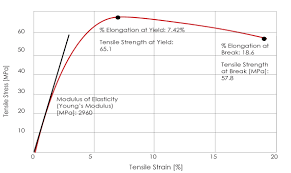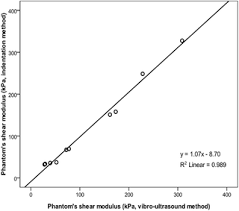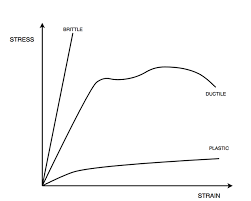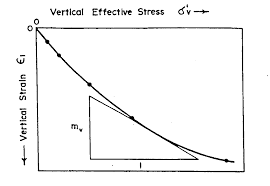## How to Calculate and Solve for Bulk Modulus of Elasticity (E) | Rock MechanicsThe image above represents bulk modulus of elasticity (E).

To compute for bulk modulus of elasticity (E), two essential parameters are needed and these parameters are young’s modulus (E) and Poisson’s ratio (v).

The formula for calculating the bulk modulus of elasticity (E):

k = E / 3(1 – 2v)

Where:

k = Bulk Modulus of Elasticity (E)
E = Young’s Modulus
v = Poisson’s Ratio

Let’s solve an example;
Find the bulk modulus of elasticity (E) when the young’s modulus is 66 and the Poisson’s ratio is 58.

This implies that;

E = Young’s Modulus = 66
v = Poisson’s Ratio = 58

k = E / 3(1 – 2v)
k = 66 / 3(1 – 2(58))
k = 66 / 3(1 – 116)
k = 66 / 3(-115)
k = 66 / -345
k = -0.19

Therefore, the bulk modulus of elasticity (E) is -0.19.

Calculating the Young’s Modulus when the Bulk Modulus of Elasticity (E) and the Poisson’s Ratio is Given.

E = k (3 – 6v)

Where:

E = Young’s Modulus
k = Bulk Modulus of Elasticity (E)
v = Poisson’s Ratio

Let’s solve an example;
Find the young’s modulus when the bulk modulus of elasticity (E) is 22 and the Poisson’s ratio is 18.

This implies that;

k = Bulk Modulus of Elasticity (E) = 22
v = Poisson’s Ratio = 18

E = k (3 – 6v)
E = 22 (3 – 6(18))
E = 22 (3 – 108)
E = 22 (-105)
E = -2376

Therefore, the young’s modulus is -2376.

## How to Calculate and Solve for Shear Modulus | Rock MechanicsThe image above represents shear modulus.

To compute for shear modulus, two essential parameters are needed and these parameters are young’s modulus (E) and Poisson’s ratio (v).

The formula for calculating the shear modulus:

G = E / 2(1 + v)

Where:

G = Shear Modulus
E = Young’s Modulus
v = Poisson’s Ratio

Let’s solve an example;
Find the shear modulus when the young’s modulus is 32 and the Poisson’s ratio is 24.

This implies that;

E = Young’s Modulus = 32
v = Poisson’s Ratio = 24

G = E / 2(1 + v)
G = 32 / 2(1 + 24)
G = 32 / 2(25)
G = 32 / 50
G = 0.64

Therefore, the shear modulus is 0.64.

Calculating the Young’s Modulus when the Shear Modulus and the Poisson’s Ratio is Given.

E = G (2 + 2v)

Where:

E = Young’s Modulus
G = Shear Modulus
v = Poisson’s Ratio

Let’s solve an example;
Find the young’s modulus when the shear modulus is 12 and the Poisson’s ratio is 10.

This implies that;

G = Shear Modulus = 12
v = Poisson’s Ratio = 10

E = G (2 +2v)
E = 12 (2 + 2(10))
E = 12 (2 + 20)
E = 12 (22)
E = 264

Therefore, the young’s modulus is 264.

## How to Calculate and Solve for Young’s Modulus | Rock MechanicsThe image above represents young’s modulus.

To compute for young’s modulus, two essential parameters are needed and these parameters are axial stress (σa) and axial strain (εa).

The formula for calculating the young’s modulus:

E = σa / εa

Where:

E = Young’s Modulus
σa = Axial Stress
εa = Axial Strain

Let’s solve an example;
Given that the axial stress is 65 and the axial strain is 45. Calculate for the young’s modulus?

This implies that;

σa = Axial Stress = 65
εa = Axial Strain = 45

E = σa / εa
E = 65 / 45
E = 1.44

Therefore, the young’s modulus is 1.44.

Calculating the Axial Stress when the Young’s Modulus and the Axial Strain is Given.

σa = E x εa

Where;

σa = Axial Stress
E = Young’s Modulus
εa = Axial Strain

Let’s solve an example;
Given that the young’s modulus is 25 and the axial strain is 10. Calculate for the axial stress?

This implies that;

E = Young’s Modulus = 25
εa = Axial Strain = 10

σa = E x εa
σa = 25 x 10
σa = 250

Therefore, the axial stress is 250.

## How to Calculate and Solve for Vertical Strain | Rock MechanicsThe image above represents vertical strain.

To compute for vertical strain, five essential parameters are needed and these parameters are Vertical Stress (σv), Poisson’s Ratio (v), Principal Horizontal Stress Component 1 (σH1), Principal Horizontal Stress Component 2 (σH2) and Young’s Modulus (E).

The formula for calculating the vertical strain:

εv = v – vσH1 – vσH2) / E

Where:

εv = Vertical Strain
σv = Vertical Stress
v = Poisson’s Ratio
σH1 = Principal Horizontal Stress Component 1
σH2 = Principal Horizontal Stress Component 2
E = Young’s Modulus

Let’s solve an example;
Find the vertical strain with a vertical stress of 44, Poisson’s ratio of 5, principal horizontal stress component 1 of 6, principal horizontal stress component 2 of 4 and young’s modulus of 12.

This implies that;

σv = Vertical Stress = 44
v = Poisson’s Ratio = 5
σH1 = Principal Horizontal Stress Component 1 = 6
σH2 = Principal Horizontal Stress Component 2 = 4
E = Young’s Modulus = 12

εv = v – vσH1 – vσH2) / E
εv = (44 – 5(6) – 5(4)) / 12
εv = (44 – 30 – 20) / 12
εv = -6 / 12
εv = -0.5

Therefore, the vertical strain is -0.5.

Calculating the Vertical Stress when the Vertical Strain, Poisson’s Ratio, Principal Horizontal Stress Component 1, Principal Horizontal Stress Component 2 and Young Modulus is Given.

σv = (εv x E) + vσH1 + vσH2

Where:

σv = Vertical Stress
εv = Vertical Strain
v = Poisson’s Ratio
σH1 = Principal Horizontal Stress Component 1
σH2 = Principal Horizontal Stress Component 2
E = Young’s Modulus

Let’s solve an example;
Find the vertical stress with a vertical strain of 52, Poisson’s ratio of 12, principal horizontal stress component 1 of 15, principal horizontal stress component 2 of 10 and young’s modulus of 13.

This implies that;

εv = Vertical Strain = 52
v = Poisson’s Ratio = 12
σH1 = Principal Horizontal Stress Component 1 = 15
σH2 = Principal Horizontal Stress Component 2 = 10
E = Young’s Modulus = 13

σv = (εv x E) + vσH1 + vσH2
σv = (52 x 13) + 12(15) + 15(10)
σv = 676 + 180 + 150
σv = 1006

Therefore, the vertical stress is 1006.

Calculating the Poisson’s Ratio when the Vertical Strain, Vertical Stress, Principal Horizontal Stress Component 1, Principal Horizontal Stress Component 2 and Young Modulus is Given.

v = σv – (εv x E) / σH1 + σH2

Where:

v = Poisson’s Ratio
εv = Vertical Strain
σv = Vertical Stress
σH1 = Principal Horizontal Stress Component 1
σH2 = Principal Horizontal Stress Component 2
E = Young’s Modulus

Let’s solve an example;
Find the Poisson’s ratio with a vertical strain of 16, vertical stress of 110, principal horizontal stress component 1 of 7, principal horizontal stress component 2 of 13 and young’s modulus of 6.

This implies that;

εv = Vertical Strain = 16
σv = Vertical Stress = 110
σH1 = Principal Horizontal Stress Component 1 = 7
σH2 = Principal Horizontal Stress Component 2 = 13
E = Young’s Modulus = 6

v = σv – (εv x E) / σH1 + σH2
v = 110 – (16 x 6) / 7 + 13
v = 110 – 96 / 20
v = 14 / 20
v = 0.7

Therefore, the Poisson’s ratio is 0.7.